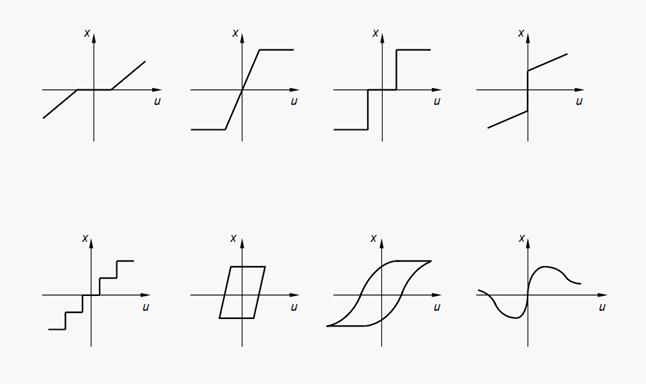Contents >> Engineering Mathematics >> Control Systems >> Dynamic Analysis of Control System of Hydraulic Drive >> Introduction

 Automatic control systems - Introduction Introduction Dynamic analysis is the important stage at designing systems of various machines and mechanisms (cars, self-propelled chassis, tractors, building, road, mountain, agricultural machines, machine tools, flying devices, stands, etc.) and allows to simulate long before creation of a pre-production model working processes and to obtain necessary data about dynamic properties of projected system and features of course of working processes, to pay attention to presence of bottlenecks in system, and before carrying out of tests to carry out optimization of the design decision on a basis of results of mathematical simulation and the alternative analysis. Mathematical simulation of various modern technical systems of the certain class (mechanical, hydraulic, electric, etc.) is connected with construction of the models providing the formalized description of any such system of arbitrary structure. For construction of formal dynamic model of a control system the method based on representation of system in the form of final element-node structure is used. In a basis of such approach the idea of a method of finite elements lays, when the complex system on a configuration can be divided conditionally into separate functional elements which mathematical description it is known and for which within limits of considered system it is possible to define unequivocally conditions of communications of these elements with each other ( input – output ). Then for the description of system as a whole it is enough to specify a name (the identifier of type) of element , to number its nodes on an input and an output , to set necessary physical, geometrical and design data (constants) and to write down the equations, transforming variables on an input of an element in variables on an output. For simulation of dynamics of control systems and regulation of the various physical nature as base elements it is possible to use linear  and nonlinear  dynamic links . Nonlinearity in control systems of technical systems usually is display of their natural physical properties. Static and dynamic types of nonlinearity are distinguished . Static nonlinearity (Fig.1) is nonlinearity of static characteristics (for example, a dry friction, a neutral zone, a clearance, a hysteresis, saturation, various nonlinear functions).Fig. 1. Some kinds of static nonlinear function Dynamic nonlinearity is connected with nonlinear differential equations of dynamics of a link. The following physical properties and parameters relate to them: nonlinear viscous friction, flow characteristics of throttles, valves and directional control valves, external characteristics of diesel engine, hydraulic clutch, hydraulic torque converter, a curve of wheel slipping, etc. All these kinds of nonlinearities are in detail considered in sections « Mobile machines », « Hydraulic systems », « Drives and transmissions ». In the given section we’ll be limited to consideration of linear dynamic links of automatic control systems (ACS) . The structure of any arbitrary dynamic system can be described by means of identification of elements, numbering of nodes (points of connection of elements in the circuit diagram by a principle an input – an output ) and formations on a basis of it matrices of communications reflecting structure (topology) of the scheme. Thus, for simulation of dynamic processes in control systems of arbitrary structure it is necessary to have: – algorithm of the structural description of arbitrary schemes; – library of mathematical models of base elements (dynamic links of ACS); – regimentation of initial data, and way of their formation and preparation; – algorithm of automatic forming of system of equations describing ACS of arbitrary structure as a whole; – method of the solution of the generated system of equations; – program realization of dynamic analysis of arbitrary schemes; – way of representation and the analysis of received results. The program analyzes the initial information and depending on set of elements and structures of investigated system, chooses from library of mathematical models of base elements the necessary equations, forming the general mathematical model of system and solving it at given external influences.

 < Previous Contents Next >